College Algebra with Corequisite Support

# Chapter 4

College Algebra with Corequisite SupportChapter 4

### 4.1Linear Functions

1.

$m= 4−3 0−2 = 1 −2 =− 1 2 ; m= 4−3 0−2 = 1 −2 =− 1 2 ;$ decreasing because $m<0. m<0.$

2.
$m= 1,868−1,442 2,012−2,009 = 426 3 =142 people per year m= 1,868−1,442 2,012−2,009 = 426 3 =142 people per year$
3.

$y=−7x+3 y=−7x+3$

4.

$H( x )=0.5x+12.5 H( x )=0.5x+12.5$

5.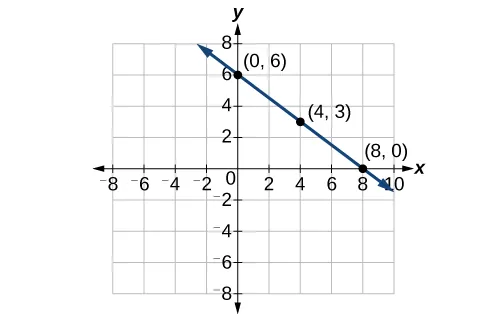6.

Possible answers include $(−3,7), (−3,7),$ $(−6,9), (−6,9),$ or $(−9,11). (−9,11).$

7.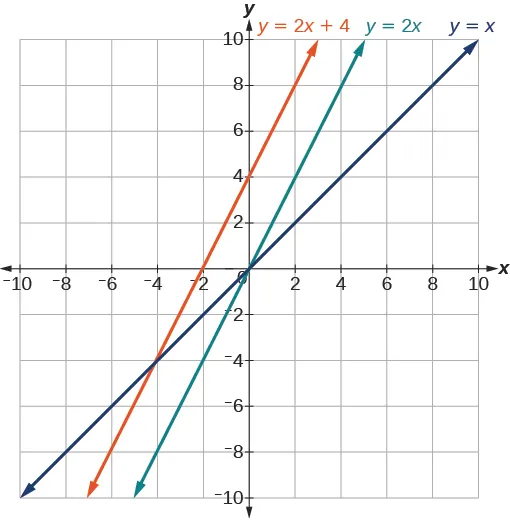8.

$( 16,0 ) ( 16,0 )$

9.
1. $f(x)=2x; f(x)=2x;$
2. $g(x)=− 1 2 x g(x)=− 1 2 x$
10.

$y=– 1 3 x+6 y=– 1 3 x+6$

### 4.2Modeling with Linear Functions

1.

$C( x )=0.25x+25,000 C(x)=0.25x+25,000$
The y-intercept is $(0,25,000) (0,25,000)$. If the company does not produce a single doughnut, they still incur a cost of $25,000. 2. 41,100 2020 3. 21.57 miles ### 4.3Fitting Linear Models to Data 2. $54°F 54°F$ 3. 150.871 billion gallons; extrapolation ### 4.1 Section Exercises 1. Terry starts at an elevation of 3000 feet and descends 70 feet per second. 3. $d( t )=100−10t d( t )=100−10t$ 5. The point of intersection is $( a,a ). ( a,a ).$ This is because for the horizontal line, all of the $y y$ coordinates are $a a$ and for the vertical line, all of the $x x$ coordinates are $a. a.$ The point of intersection is on both lines and therefore will have these two characteristics. 7. Yes 9. Yes 11. No 13. Yes 15. Increasing 17. Decreasing 19. Decreasing 21. Increasing 23. Decreasing 25. 2 27. –2 29. $y= 3 5 x−1 y= 3 5 x−1$ 31. $y=3x−2 y=3x−2$ 33. $y=− 1 3 x+ 11 3 y=− 1 3 x+ 11 3$ 35. $y=−1.5x−3 y=−1.5x−3$ 37. perpendicular 39. parallel 41. $f(0)=−(0)+2 f(0)=2 y−int:(0,2) 0=−x+2 x−int:(2,0) f(0)=−(0)+2 f(0)=2 y−int:(0,2) 0=−x+2 x−int:(2,0)$ 43. $h(0)=3(0)−5 h(0)=−5 y−int:(0,−5) 0=3x−5 x−int:( 5 3 ,0 ) h(0)=3(0)−5 h(0)=−5 y−int:(0,−5) 0=3x−5 x−int:( 5 3 ,0 )$ 45. $−2x+5y=20 −2(0)+5y=20 5y=20 y=4 y−int:(0,4) −2x+5(0)=20 x=−10 x−int:(−10,0) −2x+5y=20 −2(0)+5y=20 5y=20 y=4 y−int:(0,4) −2x+5(0)=20 x=−10 x−int:(−10,0)$ 47. Line 1: m = –10 Line 2: m = –10 Parallel 49. Line 1: m = –2 Line 2: m = 1 Neither 51. 53. $y=3x−3 y=3x−3$ 55. $y=− 1 3 t+2 y=− 1 3 t+2$ 57. 0 59. $y=− 5 4 x+5 y=− 5 4 x+5$ 61. $y=3x−1 y=3x−1$ 63. $y=−2.5 y=−2.5$ 65. F 67. C 69. A 71.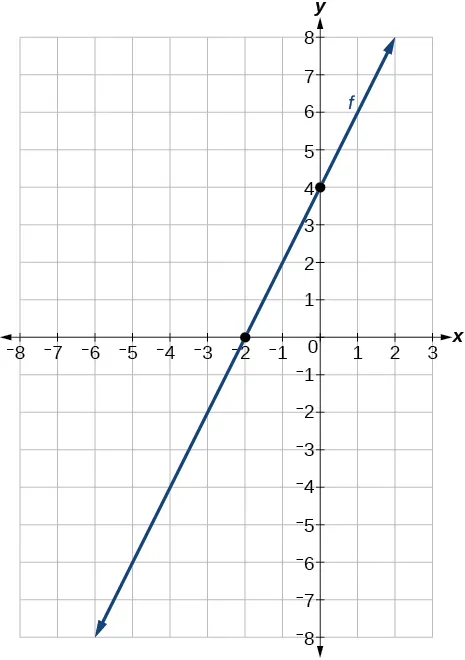73.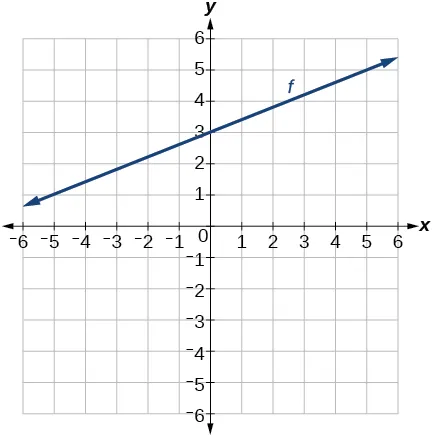75.77.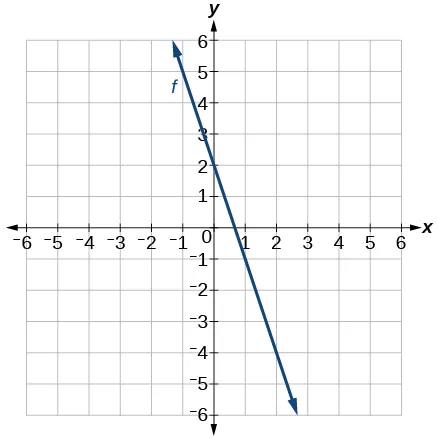79.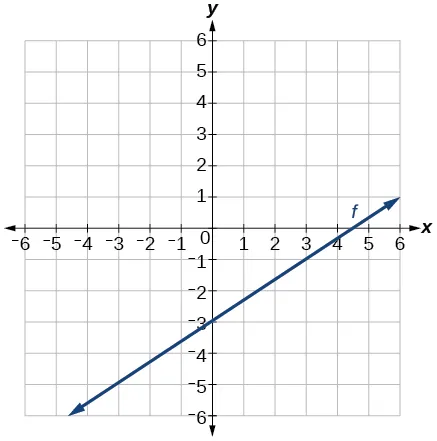81.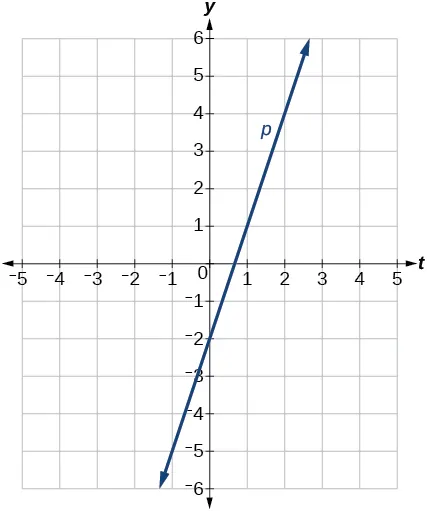83.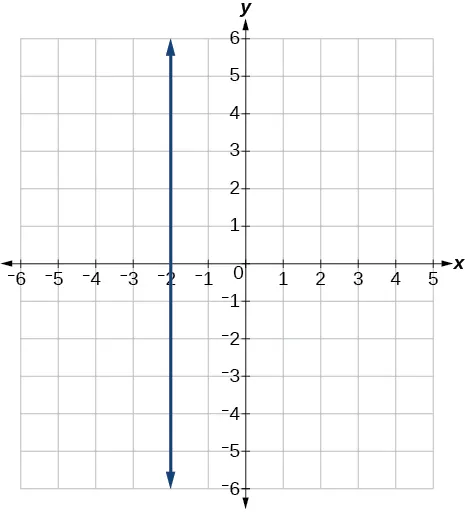85. $y=3 y=3$ 87. $x=−3 x=−3$ 89. Linear, $g(x)=−3x+5 g(x)=−3x+5$ 91. Linear, $f(x)=5x−5 f(x)=5x−5$ 93. Linear, $g(x)=− 25 2 x+6 g(x)=− 25 2 x+6$ 95. Linear, $f(x)=10x−24 f(x)=10x−24$ 97. $f(x)=−58x+17.3 f(x)=−58x+17.3$ 99.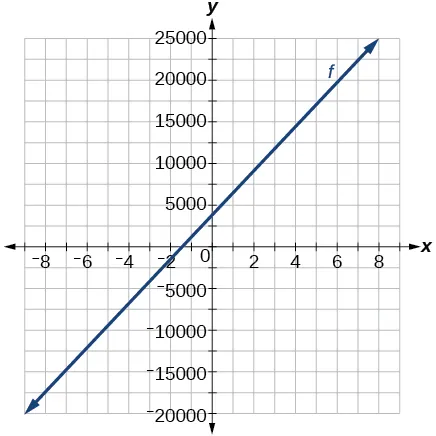101. 1. $a=11,900, b=1000.1a=11,900, b=1000.1$ 2. $q(p)=1000p –100q(p)=1000p –100$ 103.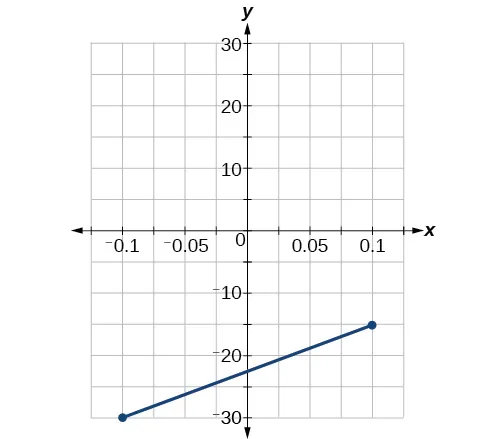105. $y=− 16 3 y=− 16 3$ 107. $x=ax=a$ 109. $y= d c–a x– ad c–a y= d c–a x– ad c–a$ 111. $y=100x–98y=100x–98$ 113. $x< 1999 201 , x> 1999 201 x< 1999 201 , x> 1999 201$ 115.$45 per training session.

117.

The rate of change is 0.1. For every additional minute talked, the monthly charge increases by $0.1 or 10 cents. The initial value is 24. When there are no minutes talked, initially the charge is$24.

119.

The slope is –400. this means for every year between 1960 and 1989, the population dropped by 400 per year in the city.

121.

C

### 4.2 Section Exercises

1.

Determine the independent variable. This is the variable upon which the output depends.

3.

To determine the initial value, find the output when the input is equal to zero.

5.

6 square units

7.

20.01 square units

9.

2,300

11.

64,170

13.

$P( t )=75,000+2500t P( t )=75,000+2500t$

15.

(–30, 0) Thirty years before the start of this model, the town had no citizens. (0, 75,000) Initially, the town had a population of 75,000.

17.

Ten years after the model began

19.

$W(t)=0.5t+7.5 W(t)=0.5t+7.5$

21.

$( −15,0 ) ( −15,0 )$ : The x-intercept is not a plausible set of data for this model because it means the baby weighed 0 pounds 15 months prior to birth. $( 0,7.5 ) ( 0,7.5 )$ : The baby weighed 7.5 pounds at birth.

23.

At age 5.8 months

25.

$C( t )=12,025−205t C( t )=12,025−205t$

27.

$( 58.7,0 ): ( 58.7,0 ):$ In roughly 59 years, the number of people inflicted with the common cold would be 0. $( 0,12,025 ) ( 0,12,025 )$ Initially there were 12,025 people afflicted by the common cold.

29.

2063

31.

$y=−2t+180 y=−2t+180$

33.

In 2070, the company’s profit will be zero.

35.

$y=30t−300 y=30t−300$

37.

(10, 0) In the year 1990, the company’s profits were zero

39.

Hawaii

41.

During the year 1933

43.

$105,620 45. 1. 696 people 2. 4 years 3. 174 people per year 4. 305 people 5. P(t) = 305 + 174t 6. 2,219 people 47. 1. C(x) = 0.15x + 10 2. The flat monthly fee is$10 and there is a $0.15 fee for each additional minute used 3.$113.05
49.

P(t) = 190t + 4,360

51.
1. 5.5 billion cubic feet
2. During the year 2017
53.

More than 133 minutes

55.

More than $42,857.14 worth of jewelry 57. More than$66,666.67 in sales

### 4.3 Section Exercises

1.

When our model no longer applies, after some value in the domain, the model itself doesn’t hold.

3.

We predict a value outside the domain and range of the data.

5.

The closer the number is to 1, the less scattered the data, the closer the number is to 0, the more scattered the data.

7.

61.966 years

9.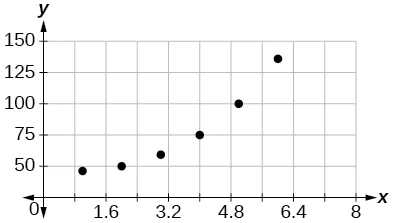No.

11.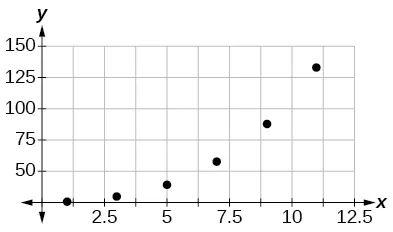No.

13.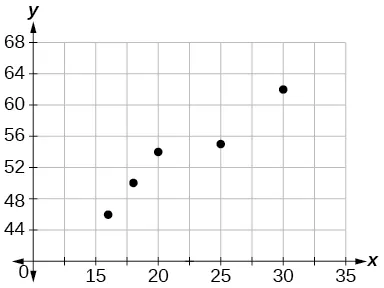Interpolation. About $60°F. 60°F.$

15.

17.

19.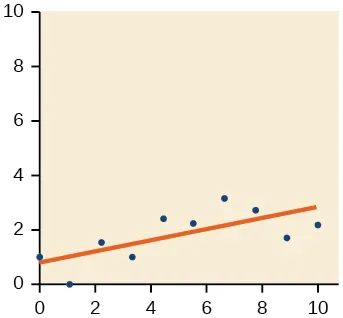21.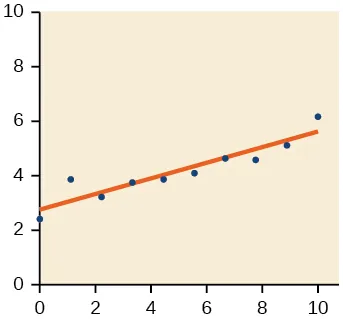23.

Yes, trend appears linear because $r=0.985 r=0.985$ and will exceed 12,000 near midyear, 2016, 24.6 years since 1992.

25.

$y=1.640x+13.800, y=1.640x+13.800,$ $r=0.987 r=0.987$

27.

29.

$y=−1.981x+60.197; y=−1.981x+60.197;$ $r=−0.998 r=−0.998$

31.

$y=0.121x−38.841,r=0.998 y=0.121x−38.841,r=0.998$

33.

$(−2,−6),(1,−12),(5,−20),(6,−22),(9,−28); (−2,−6),(1,−12),(5,−20),(6,−22),(9,−28);$ Yes, the function is a good fit.

35.

$(189.8,0) (189.8,0)$ If 18,980 units are sold, the company will have a profit of zero dollars.

37.

$y=0.00587x+1985.41 y=0.00587x+1985.41$

39.

$y=20.25x−671.5 y=20.25x−671.5$

41.

$y=−10.75x+742.50 y=−10.75x+742.50$

### Review Exercises

1.

Yes

3.

Increasing

5.

$y=−3x+26 y=−3x+26$

7.

3

9.

$y=2x−2 y=2x−2$

11.

Not linear.

13.

parallel

15.

$(–9,0);(0,–7) (–9,0);(0,–7)$

17.

Line 1: $m=−2; m=−2;$ Line 2: $m=−2; m=−2;$ Parallel

19.

$y=−0.2x+21 y=−0.2x+21$

21.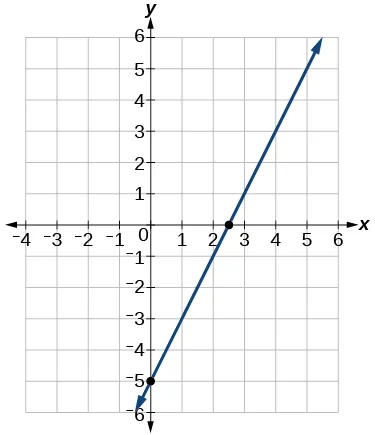23.

More than 250

25.

118,000

27.

$y=−300x+11,500 y=−300x+11,500$

29.
1. 800
2. 100 students per year
3. $P( t )=100t+1700 P( t )=100t+1700$
31.

18,500

33.

\$91,625

35.

Extrapolation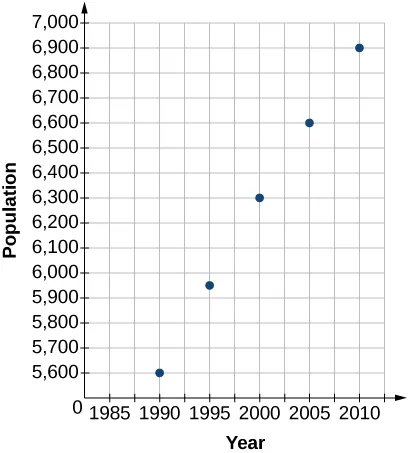37.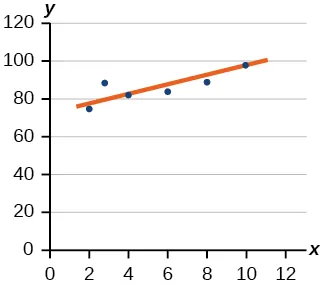39.

2023

41.

43.

2027

45.

7,660

### Practice Test

1.

Yes

3.

Increasing

5.

y = −1.5x − 6

7.

y = −2x − 1

9.

No

11.

Perpendicular

13.

(−7, 0); (0, −2)

15.

y = −0.25x + 12

17.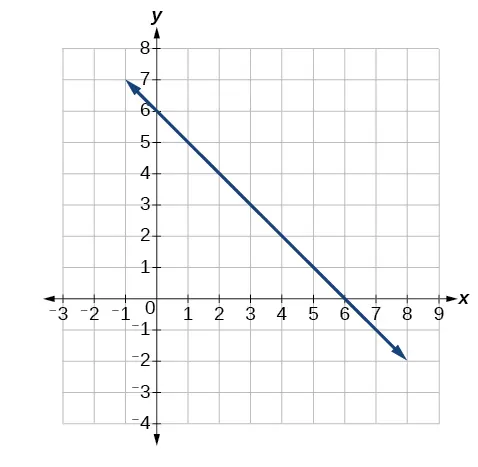Slope = −1 and y-intercept = 6

19.

150

21.

165,000

23.

y = 875x + 10,625

25.
1. 375
2. dropped an average of 46.875, or about 47 people per year
3. y = −46.875t + 1250
27.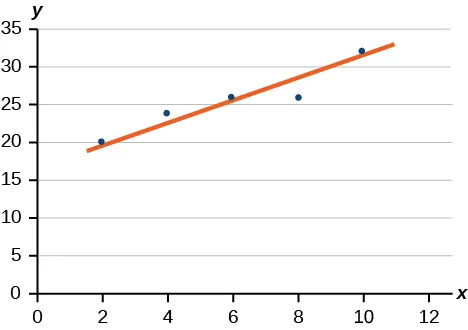29.

In early 2018

31.

y = 0.00455x + 1979.5

33.

r = 0.999